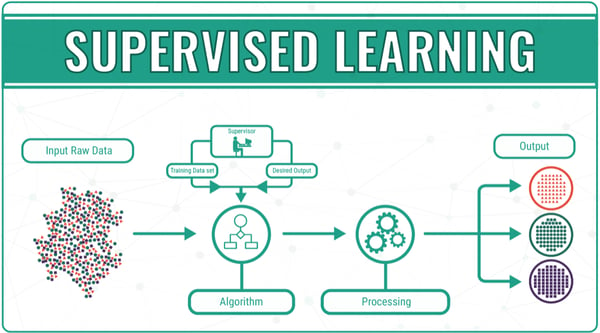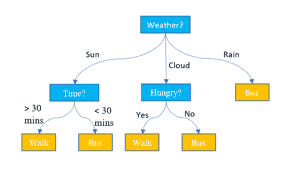Related Tags

supervised
learning
machine
algorithms

# Supervised learning algorithmsEducative Answers Team

Supervised machine learning occurs when a model is trained on correctly labeled, existing data.

Supervised learning algorithms iteratively make predictions on the training data and match these predictions with the actual label of the data. When the algorithms achieve an acceptable level of performance, the learning stops.Credits: Medium.com

There are several supervised machine learning algorithms:

• Nearest Neighbor
• Naive Bayes
• Decision Trees
• Linear Regression
• Neural Networks

## Nearest Neighbor

The K Nearest Neighbor (KNN) algorithm assumes that similar things exist in close proximity. By this principle, similar data points must be clustered close to each other. KNN captures the idea of similarity to calculate the distance between points on a graph.

## Naive Bayes

Naive Bayes classifiers are based on Bayes’ theorem and assume that the occurrence or absence of a feature does not influence the presence or absence of some other feature.

## Decision TreesA decision tree is a tree-like graph made up of decisions and their possible consequences. It is one way to display an algorithm that only contains conditional control statements.

In decision tree classification, each node is the feature of an instance that should be classified, and each branch represents a value that the node can assume.

## Linear Regression

Linear regression is used to find a linear relationship between the target and one or more predictors. It is used to explain the relationship between one dependent variable and one or more independent variables. This regression technique predicts a single output value using training data.

## Neural NetworksA simple neural network consists of interconnected neurons transferring information to each other. Each neuron multiplies its input with its weight(s), applies the activation function on the result, and passes its output on to other neurons. With the help of examples in the training process, a neural network adjusts its weights such that it correctly classifies an unseen input.

RELATED TAGS

supervised
learning
machine
algorithms